Ex 5.2

Chapter 5 Class 11 Complex Numbers (Term 1)
Serial order wise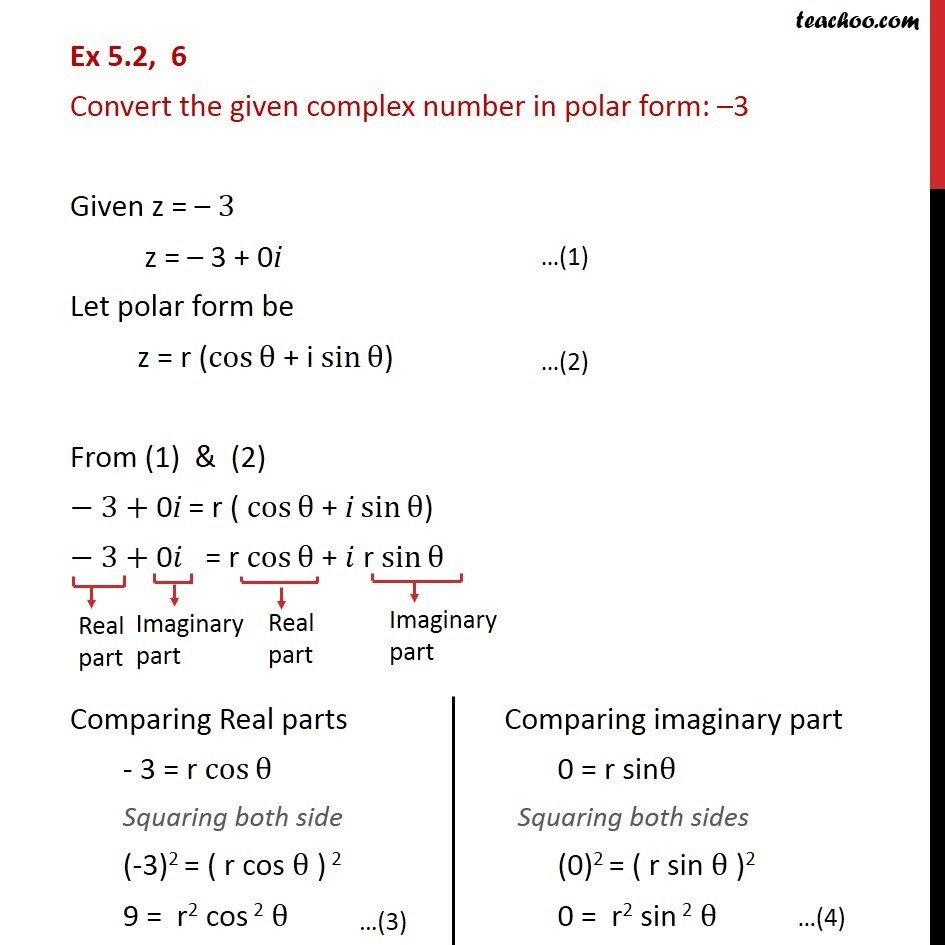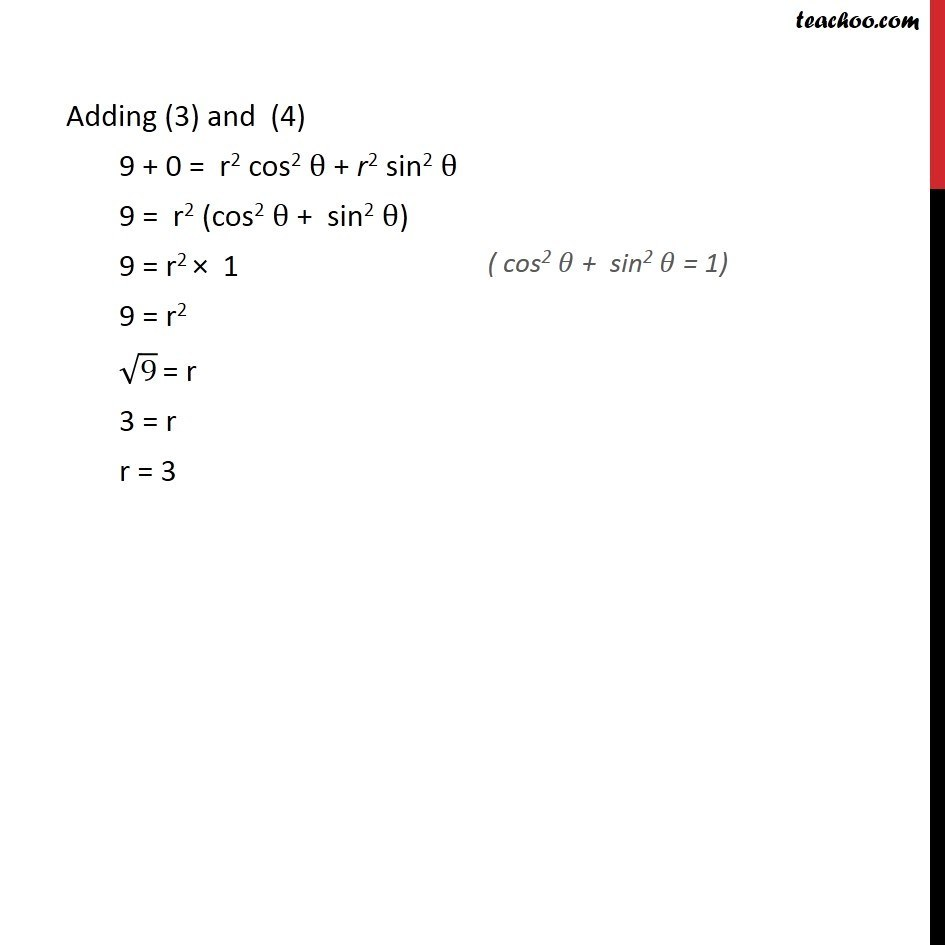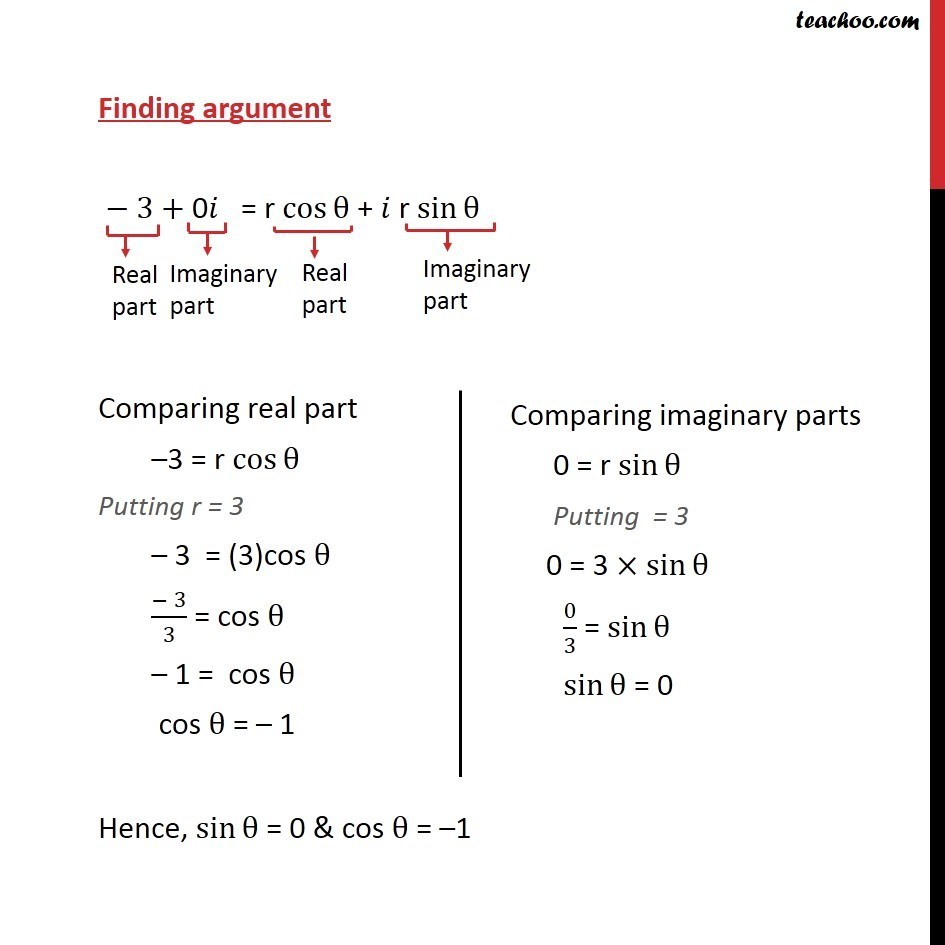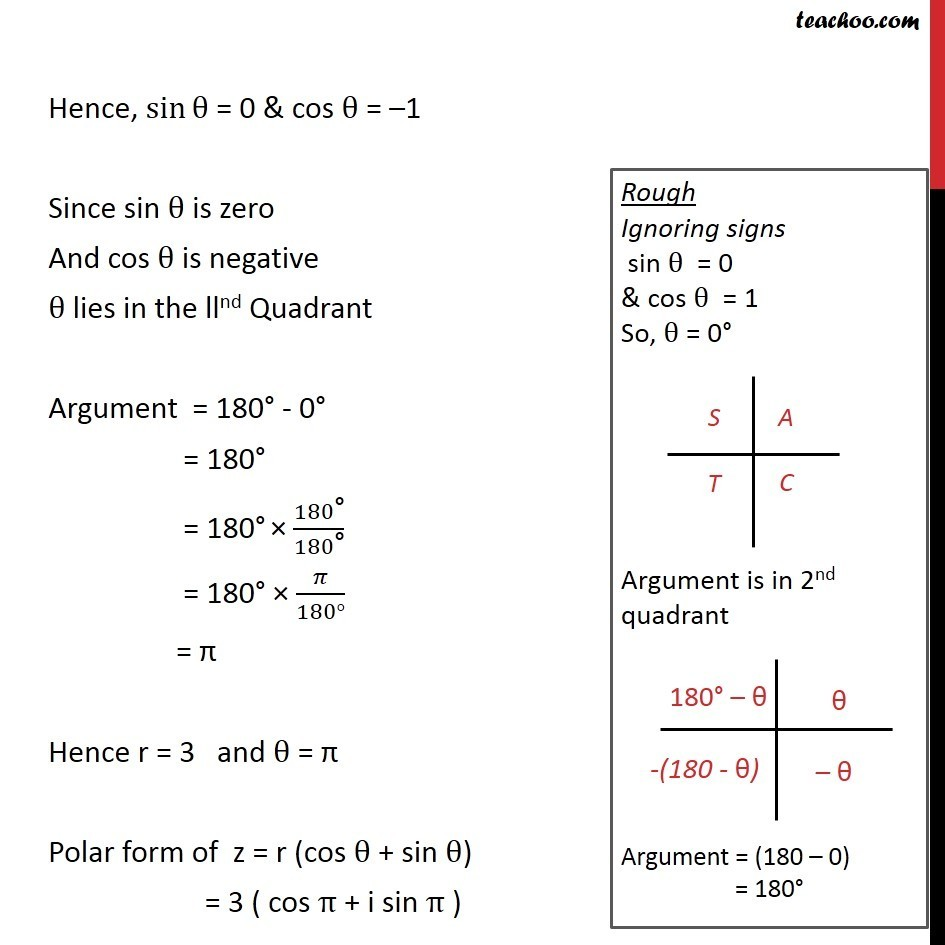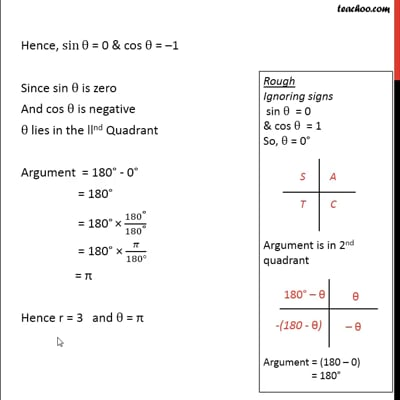This video is only available for Teachoo black users

### Transcript

Ex 5.2, 6 Convert the given complex number in polar form: –3 Given z = – 3 z = – 3 + 0i Let polar form be z = r (cos⁡θ + i sin⁡θ) From (1) & (2) − 3+ 0i = r ( cos⁡θ + i sin⁡θ) − 3+ 0𝑖 = r〖 cos〗⁡θ + 𝑖 r sin⁡θ Adding (3) and (4) 9 + 0 = r2 cos2 θ + r2 sin2 θ 9 = r2 (cos2 θ + sin2 θ) 9 = r2 × 1 9 = r2 √9 = r 3 = r r = 3 Finding argument − 3+ 0𝑖 = r〖 cos〗⁡θ + 𝑖 r sin⁡θ Comparing real part –3 = r cos⁡θ Putting r = 3 – 3 = (3)cos θ (− 3)/3 = cos θ – 1 = cos θ cos θ = – 1 Hence, sin⁡θ = 0 & cos θ = –1 Hence, sin⁡θ = 0 & cos θ = –1 Since sin θ is zero And cos θ is negative θ lies in the llnd Quadrant Argument = 180° - 0° = 180° = 180° × 180"°" /180"°" = 180° × 𝜋/(180°) = π Hence r = 3 and θ = π Polar form of z = r (cos θ + sin θ) = 3 ( cos π + i sin π )